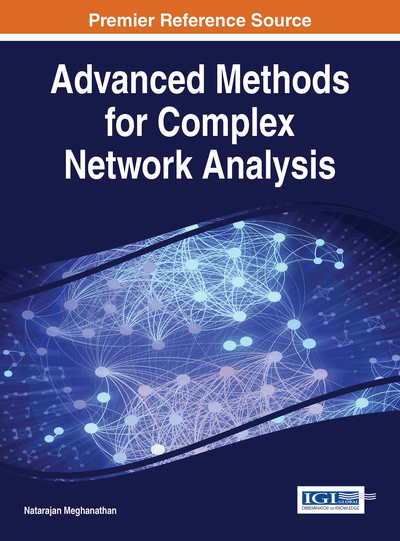# On Vertex Cover Problems in Distributed Systems

Can Umut Ileri (Ege University, Turkey), Cemil Aybars Ural (Ege University, Turkey), Orhan Dagdeviren (Ege University, Turkey) and Vedat Kavalci (Izmir University, Turkey)
DOI: 10.4018/978-1-4666-9964-9.ch001
Available
\$33.75
List Price: \$37.50
10% Discount:-\$3.75
TOTAL SAVINGS: \$3.75

## Abstract

An undirected graph can be represented by G(V,E) where V is the set of vertices and E is the set of edges connecting vertices. The problem of finding a vertex cover (VC) is to identify a set of vertices VC such that at least one endpoint of every edge in E is incident to a vertex V in VC. Vertex cover is a very important graph theoretical structure for various types of communication networks such as wireless sensor networks, since VC can be used for link monitoring, clustering, backbone formation and data aggregation management. In this chapter, we will define vertex cover and related problems with their applications on communication networks and we will survey some important distributed algorithms on this research area.
Chapter Preview
Top

## Introduction

In this section the vertex cover problems and the contributions of this chapter will be introduced.

### Vertex Cover Problems

Given a graph G(V, E) where V is the set of vertices and E is the set of edges between vertices, the problem to find a set of vertices VC ∈ V such that for any edge {u,v} ∈ E, at least one of u and v is in VC is called vertex cover problem. V itself is a vertex cover and it may have numerous subsets satisfying the vertex cover conditions. Among all possible vertex covers of a given graph, the one(s) that have the minimum cardinality are called the minimum vertex cover. Finding the minimum vertex cover on an undirected graph is an NP-Hard problem. A minimal vertex cover is a vertex cover VC whose cardinality cannot be decreased, in other words, exclusion of any vertex from VC would break the vertex cover conditions. Note that a minimal vertex cover is not required to have the minimum possible cardinality. Figure 1 illustrates such a case.

Figure 1.

Minimal and Minimum Vertex Covers: Dark vertices are the ones in the VC solution set. Removal of any vertex from the VC solution in (b) breaks the VC conditions, that is, the solution is minimal. However its cardinality is 6, while there is a possible solution having less number of vertices (5) as shown in (c)

Several objective functions are used for vertex cover problem. In minimum cardinality vertex cover problem, the optimum vertex cover VC* is the one (or possible ones) that has (or have) the minimum cardinality among all possible vertex covers. In a weighted graph where a weight wv is assigned to each vertex v ∈ V, the objective could be to find a vertex cover VC* in which the total weights of the vertices are the minimum among all possible vertex covers of the graph. This problem is called minimum weighted vertex cover problem and is also NP-Hard. Note that the vertex cover having the minimum weight may not be the one having the minimum cardinality. Figure 2 illustrates such a case.

Numbers on the vertices are the weights. In (a), solution size is 3 (the minimum cardinality) and total weight is 23; while in (b), total weight is only 19 (minimum weight), although the cardinality is doubled.

Another variation of the problem is the connected vertex cover problem in which the graph induced by the vertex cover is connected. Vertex cover can be used for link monitoring to provide secure communication in wireless ad hoc networks. To achieve this operation, nodes in VC set are trusted and can monitor the transmissions between nodes. Since every communication link will be under the coverage of one or more nodes. Other important applications are data aggregation management, backbone and cluster formations, hub or router location designation and traffic control on the information flow.

## Complete Chapter List

Search this Book:
Reset